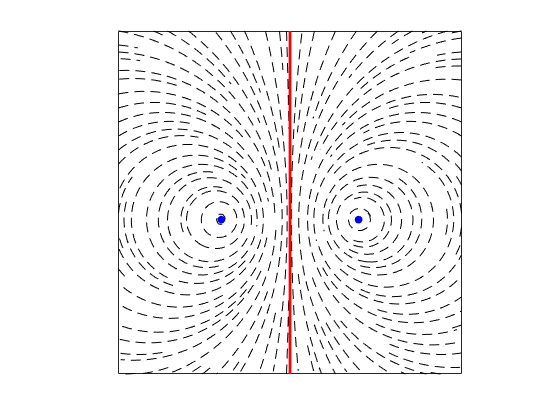Categories

# Conformal Mapping: Point Vortex Near a Corner

I wanted to know what a point vortex would do near a corner with an arbitrary angle. Time to dust off the complex analysis! A really useful summary of using complex analysis to model potential flow is here.

I will use the method of images to ensure that there is no flow through the wall, then conformally map the flow to turn it into a corner.

Define the initial complex plane as $c= a+i b$. Here, we have a vertical wall on the imaginary axis, and a vortex at $c_v = a_v+i b_v$ with strength $\Gamma$. To ensure that there is no flow through the wall, place an image vortex at $c_I = -a_v+i b_v=-\overline{c_v}$ with strength $-\Gamma$, using the definition of the complex conjugate. The complex potential, F, and complex velocity, W, are:

$F_c = \frac{\Gamma}{2 \pi i} \left( \log(c-c_v)-\log(c-c_I) \right)$
$W_c = \frac{\Gamma}{2 \pi i} \frac{c_v-c_I}{(c-c_v)(c-c_I)}$.

The streamlines around the vertical-wall flow look like:The wall is in red, the vortex is on the right, and the image vortex is on the left.

Next, I want to transform the space so that it gives a corner at the origin. The walls emerge at angle $\pm \alpha$. The new complex plane is $z= x+i y = c^{2 \alpha/\pi}=c^k$, with $k=\pi/(2 \alpha)$. The case where $\alpha = \pi/2$ (or $k=1$) is equal to the vertical wall case. Smaller values of $k$ give an external corner, or internal with larger values. This transformation is applied to the potential, giving

$F = \frac{\Gamma}{2 \pi i} \left( \log(z^k-z_v^k)-\log(z^k-z_I^k) \right)$
$W = \frac{\Gamma k}{2 \pi i} \frac{z^{k-1}(z^k-z_I^k)}{(z^k-z_v^k)(z^k-z_I^k)}$.

Let’s look at the result with internal and external corners. Note that the branch cuts are chosen such that the velocity to the left of the wall is non-physical.

Neat. The flow bends around the wall, as it should.

Next question: how does the vortex move? What are its pathlines? How fast does it go? For this, let’s say that the motion of the vortex is defined by the velocity field created by the image vortex. So we evaluate the velocity from the image vortex, at the position of the main vortex. Additionally, define $z_v=r_v e^{i \theta_v}$, and we can make some simplifications:

\begin{aligned} F_{IV} &= \frac{-\Gamma}{2 \pi i}\log \left( z_v^k+\overline{z_v^k} \right) \\ &= \frac{-\Gamma}{2 \pi i}\log \left( r_v^k e^{i k\theta_v}+r_v^k e^{-i k\theta_v} \right) \\ &= \frac{-\Gamma}{2 \pi i}\log \left( 2 r_v^k \cos(k \theta_v) \right) \\ W_{IV} &= \frac{-\Gamma k}{2 \pi i} \frac{z_v^{k-1}}{z_v^k+\overline{z_v^k}} \\ &= \frac{-\Gamma k}{2 \pi i} \frac{r_v^{k-1}e^{i (k-1) \theta_v}}{r_v^k e^{i k\theta_v} +r_v^k e^{-i k\theta_v}} \\ &= \frac{-\Gamma k}{4 \pi i} \frac{e^{i (k-1) \theta_v}}{r_v \cos(k \theta_v)}\end{aligned}.

Looking at the complex potential, it is purely imaginary. That means that its isocontours are the pathlines of the vortex. A little more math finds that the pathline that passes through $z=x_0+i*0$ is

$r_v^k = \frac{x_0^k}{\cos(\theta_v k)}$.

Do they end up hitting the wall with infinite travel? The distance from the wall to the vortex (in the upper half plane) is $d= r_v \sin( \alpha-\theta_v) = x_0 \sin( \alpha-\theta_v) \cos(k \theta_v)^{1/k}$. For a vertical wall, the vortex stays at the same distance forever. For an internal corner, the vortex approaches the wall, and with external corners, it recedes from the wall.
How quickly does the vortex travel? For that, we want $|W_{IV}|$:
\begin{aligned} |W_{IV}| &= \frac{|\Gamma| \, k}{4 \pi r_v \cos(k \theta_v)} \\ &= \frac{|\Gamma|\, k r_v^{k-1} x_0^k}{4 \pi} \end{aligned}